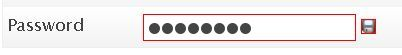## How to style input fields with CSS?

There are situations where you need to style input fields depending on there types.

Doing this is wrong:

``````input{
border:solid 1px red;
}``````

As you see in the image, the CSS rule has been applied to all input fields Continue reading “How to style input fields with CSS?”

## Maths

Ok, so you already know how to generate positive rondom numbers but let’s do it again:

To generate a random number varying from 0 to 9 we will use this code:

`var randomN = Math.floor(Math.random()*10)`

This expression on the right of the “=”

1. produces a random number from 0 to 1 (excluding 1, say 0.917) with `Math.random()`
2. multiplies it by 10 (9.71)
3. removes decimals (9) with `Math.floor`

For negative values from -9 to 0 we will  simply subtract 9 from the previous result:

`var negativeRandomN = Math.floor(Math.random()*10) - 9`

Now what if you wanted a rondom integer from -10 to 20… ?
Yes you guessed it right! we will generate a random number from 0 to 30 then substact 10 from it

`var randomN = Math.floor(Math.random()*31) - 10`

So the rule is:
To generate a random integer from m to n, here is the code

```var randomN = Math.floor(Math.random() * (n-m+1)) + m
```

## Examples

```# Random integer from 1 to 10
var randomN = Math.floor(Math.random() * 10) + 1

# Random integer from -100 to 100
var randomN = Math.floor(Math.random() * 201) -100

# Random integer from 0 to 255
var randomN = Math.floor(Math.random() * 256)
```

## What is the best free PhotoShop alternative?

The GIMP is the oldest and surely the best free open source image manipulation program, there are many forums that support it an you can find tutorials very easily.## What is email tracking?

E-mail tracking is used by individuals, e-mail marketers, spammers and phishers, to verify that e-mails are actually read by recipients, that email addresses are valid, and that the content of e-mails has made it past spam filters. Continue reading “What is email tracking?”

## On my WordPress theme, How can I find which page is being requested by the visitor?

So you want to know if the visitor is viewing the homepage, a category or a page? WordPress have easy functions for that:

• is_home() : returns true if the visitor is viewing your blog homepage
• is_category() : returns true if viewing a category archive
• is_category(‘3’) : returns true if viewing the archive of the category with the id 3
• is_category(‘People and society’): return true if viewing the archive of the category with the name People and society
• is_category(‘people-society’): return true if viewing the archive of the category with the slug people-society

These are the most used and you can find a lot more on the WordPress Codex.